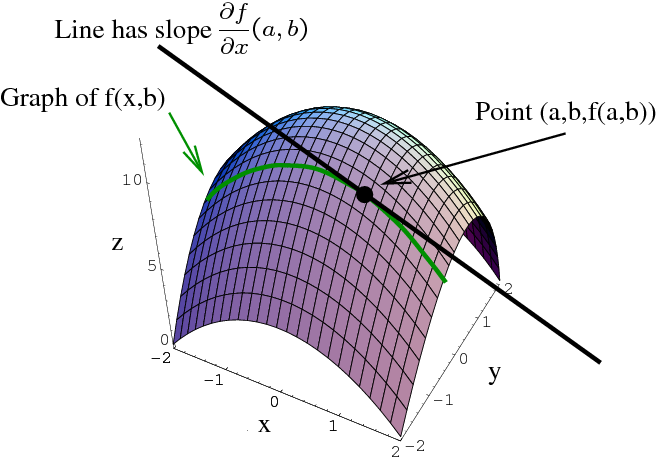# Math Insight

### Image: Partial derivative as slopeThe graph of a function $z=f(x,y)$ is a surface, and fixing $y=b$ gives a curve (shown in green). The partial derivative $\pdiff{f}{x}(a,b)$ is the slope of the tangent line to this curve at the point where $x=a$.

Image file: partial_derivative_as_slope.png